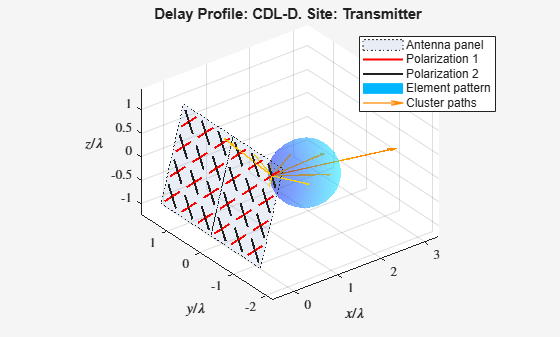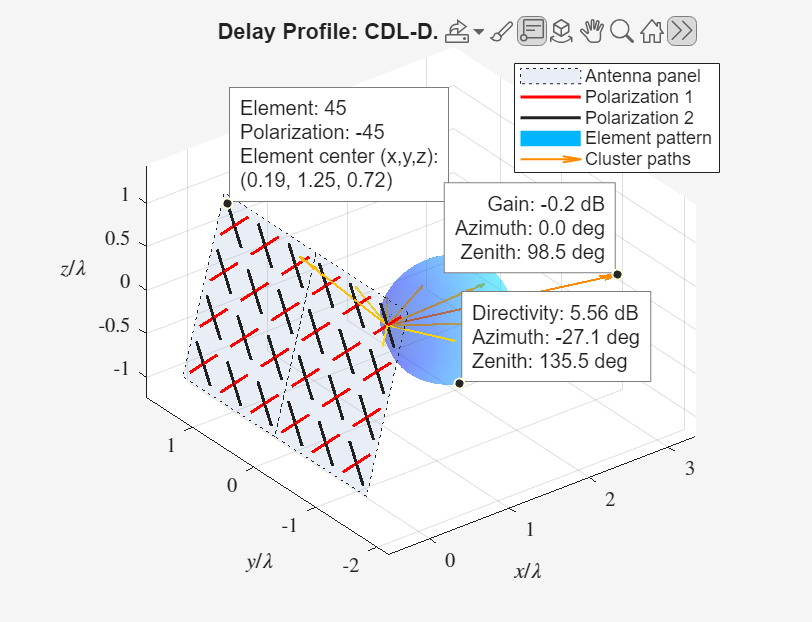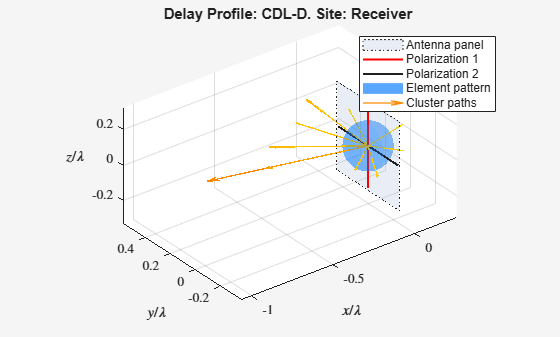# displayChannel

Visualize and explore CDL channel model characteristics

## Syntax

``fig = displayChannel(cdl)``
``fig = displayChannel(cdl,Name,Value)``

## Description

example

````fig = displayChannel(cdl)` displays geometric and electromagnetic characteristics of the specified clustered delay line (CDL) channel model at the transmitter and the receiver ends. The visualization includes the position, polarization, and directivity radiation pattern of the antenna elements, cluster paths directions, and average path gains. Because all antenna elements are equal, the visualization shows the radiation pattern of the first antenna element only and displays the cluster paths directions centered also at the first antenna element. By adding customized data tips to the visualization windows, you can explore antenna element, element pattern, and cluster paths characteristics. The function also returns an array of `figure` objects that correspond to the displayed visualization windows.```
````fig = displayChannel(cdl,Name,Value)` specifies visualization options of the displayed channel characteristics by using one or more name-value pair arguments. For example, `'LinkEnd','Tx'` specifies visualization for the transmitter end only. Unspecified options take default values.```

## Examples

collapse all

This example shows how to visualize CDL channel characteristics and explore channel information about the antenna element, element pattern, and cluster paths.

Define the channel configuration structure by using an `nrCDLChannel` System object. Specify the delay profile as CDL-D.

```cdl = nrCDLChannel; cdl.DelayProfile = 'CDL-D';```

Configure the transmit array size as a vector of the form $\left[M\text{\hspace{0.17em}}N\text{\hspace{0.17em}}P\text{\hspace{0.17em}}{\mathit{M}}_{\mathrm{g}\text{\hspace{0.17em}}}{\mathit{N}}_{\mathrm{g}}\right]=\left[4\text{\hspace{0.17em}}3\text{\hspace{0.17em}}2\text{\hspace{0.17em}}1\text{\hspace{0.17em}}2\right]$, which specifies two rectangular panels (${\mathit{M}}_{\mathrm{g}}=1$and ${\mathit{N}}_{\mathrm{g}}=2$) of a 4-by-3 antenna array ($\mathit{M}=4$ and $\mathit{N}=3$) and two polarizations ($\mathit{P}=2$). The total number of polarized elements in the array is $M×N×P×{\mathit{M}}_{\mathrm{g}}×{\mathit{N}}_{\mathrm{g}}=48$.

```txSize = [4 3 2 1 2]; cdl.TransmitAntennaArray.Size = txSize;```

Configure the vertical and horizontal element spacing and the vertical and horizontal panel spacing, in wavelength, as a vector of the form $\left[{\lambda }_{\mathrm{v}}\text{\hspace{0.17em}}{\lambda }_{\mathrm{h}}\text{\hspace{0.17em}}{\mathrm{dg}}_{\mathrm{v}}\text{\hspace{0.17em}}{\mathrm{dg}}_{\mathrm{h}}\right]$. Because panel spacing is measured from the center of the panels, to avoid panel overlapping, set ${\mathrm{dg}}_{\mathrm{h}}$ to a value greater than 1 wavelength. To ensure uniform antenna element spacing across vertically and horizontally separated panels, configure panel spacings as ${\mathrm{dg}}_{\mathrm{v}}=\text{\hspace{0.17em}}{\lambda }_{\mathrm{v}}×\mathit{M}$ and ${\mathrm{dg}}_{\mathrm{h}}={\lambda }_{\mathrm{h}}×\mathit{N}$, respectively.

```lambda_v = 0.5; lambda_h = 0.5; dg_v = lambda_v*txSize(1); % lambda_v * M dg_h = lambda_h*txSize(2); % lambda_h * N cdl.TransmitAntennaArray.ElementSpacing = [lambda_v lambda_h dg_v dg_h];```

Configure the mechanical orientation of the array as $\left[\alpha \text{\hspace{0.17em}}\beta \text{\hspace{0.17em}}\gamma {\right]}^{\mathit{T}}={\left[0\text{\hspace{0.17em}}15\text{\hspace{0.17em}}0\right]}^{\mathit{T}}$, which specifies 0 degrees bearing, 15 degrees downtilt, and 0 degrees slant.

`cdl.TransmitArrayOrientation = [0 15 0]';`

For an overview of all other transmit antenna array properties, see the TransmitAntennaArray property of the `nrCDLChannel` System object.

Display the channel characteristics at the transmitter end.

`figTx = displayChannel(cdl,'LinkEnd','Tx');`

The generated figure supports customized data tips. Add data tips in the current figure by enabling the data cursor mode.

`datacursormode on;`With data cursor mode enabled, explore channel characteristics by adding data tips. To create a data tip, click a data point. To create multiple data tips, press the Shift key while clicking the data points.

For example, this figure shows data tips added to the antenna element, element pattern, and cluster paths at the transmitter end.

• Antenna element data tips include information about the position, polarization angle, and element number of each antenna element. The element numbers indicate the order in which the channel model maps input signals column-wise to antenna elements. For more details, see the `TransmitAntennaArray.size` property of the `nrCDLChannel` System object.

• Element pattern data tips include the directivity corresponding to any azimuth and zenith angles.

• Cluster path data tips include the average path gain and azimuth and zenith angles of the cluster path.Visualize and explore channel characteristics at the receiver end. To customize the receive antenna array, use the ReceiveAntennaArray property of the `nrCDLChannel` System object. Then, display the channel characteristics at the receiver end by calling the `displayChannel` function with the` 'LinkEnd','Rx'` name-value pair argument.

`figRx = displayChannel(cdl,'LinkEnd','Rx');`

Explore channel information about the antenna element, element pattern, and cluster paths at the receiver end by enabling data cursor mode for the current figure.

`datacursormode on;`## Input Arguments

collapse all

CDL channel model, specified as an `nrCDLChannel` System object. The `nrCDLChannel` object implements the multi-input multi-output (MIMO) link-level fading channel specified in TR 38.901 Section 7.7.1.

### Name-Value Arguments

Specify optional pairs of arguments as `Name1=Value1,...,NameN=ValueN`, where `Name` is the argument name and `Value` is the corresponding value. Name-value arguments must appear after other arguments, but the order of the pairs does not matter.

Before R2021a, use commas to separate each name and value, and enclose `Name` in quotes.

Example: `'LinkEnd','Tx'` specifies visualization for the transmitter end only.

Link-level channel end, specified as the comma-separated pair consisting of `'LinkEnd'` and one of these values:

• `'Both'` — The function displays channel characteristics at both ends: the transmitter and receiver ends.

• `'Tx'` — The function displays channel characteristics only at the transmitter end.

• `'Rx'` — The function displays channel characteristics only at the receiver end.

Data Types: `char` | `string`

Polarization angle of antenna elements, specified as the comma-separated pair consisting of `'Polarization'` and `'on'` or `'off'`. When set to `'on'`, the function displays the polarization angle of the antenna elements.

Data Types: `char` | `string`

Directivity radiation pattern of antenna, specified as the comma-separated pair consisting of `'ElementPattern'` and `'on'` or `'off'`. When set to `'on'`, the function displays the directivity radiation pattern of the antenna elements.

Note

In the specified CDL channel model, `cdl`, the antenna element pattern is the same for all antenna elements. To orient the array with respect to the cluster paths, the function displays the element pattern centered at the first element of the array.

Data Types: `char` | `string`

Direction and average gain of cluster paths, specified as the comma-separated pair consisting of `'ClusterPaths'` and `'on'` or `'off'`. When set to `'on'`, the function displays the direction and average gain of the cluster paths.

Note

In the specified CDL channel model, `cdl`, the cluster path directions are the same for all antenna elements. To orient the array with respect to the cluster paths, the function displays the path directions centered at the first element of the array.

Data Types: `char` | `string`

## Output Arguments

collapse all

Visualization windows, returned as a 1-by-2 array of `figure` objects.

 3GPP TR 38.901. “Study on channel model for frequencies from 0.5 to 100 GHz.” 3rd Generation Partnership Project; Technical Specification Group Radio Access Network.